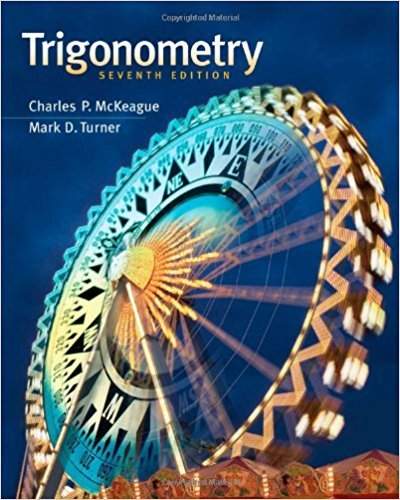×
×

# Use a calculator to find , to the nearest tenth of aISBN: 9781111826857 186

## Solution for problem 3 Chapter 1-10

Trigonometry | 7th Edition

• Textbook Solutions
• 2901 Step-by-step solutions solved by professors and subject experts
• Get 24/7 help from StudySoup virtual teaching assistantsTrigonometry | 7th Edition

4 5 1 346 Reviews
29
5
Problem 3

Use a calculator to find , to the nearest tenth of a degree, if 0 360 and sec 1.5450 with in QIV. 4. H

Step-by-Step Solution:
Step 1 of 3

Ch. 3 ➔ Appropriate measures depend on the shape ◆ Symmetric: mean and standard deviation ◆ Skewed: median and interquartile range ➔ The mean: The average ◆ Literally the balancing point ◆ For a symmetric distribution, the mean is at the center and close to...

Step 2 of 3

Step 3 of 3

##### ISBN: 9781111826857

The answer to “Use a calculator to find , to the nearest tenth of a degree, if 0 360 and sec 1.5450 with in QIV. 4. H” is broken down into a number of easy to follow steps, and 24 words. This textbook survival guide was created for the textbook: Trigonometry, edition: 7. This full solution covers the following key subjects: . This expansive textbook survival guide covers 85 chapters, and 4184 solutions. The full step-by-step solution to problem: 3 from chapter: 1-10 was answered by , our top Math solution expert on 12/27/17, 07:46PM. Trigonometry was written by and is associated to the ISBN: 9781111826857. Since the solution to 3 from 1-10 chapter was answered, more than 230 students have viewed the full step-by-step answer.

Unlock Textbook Solution